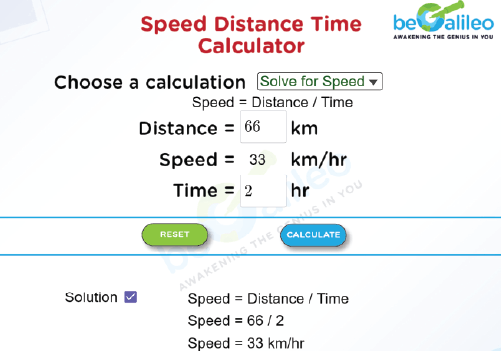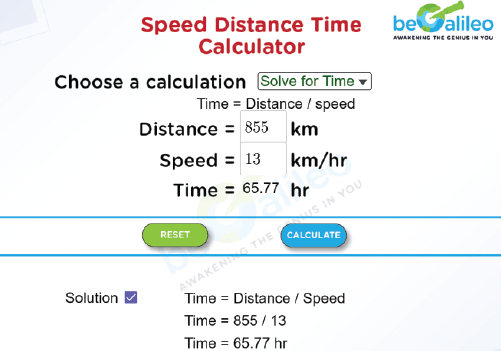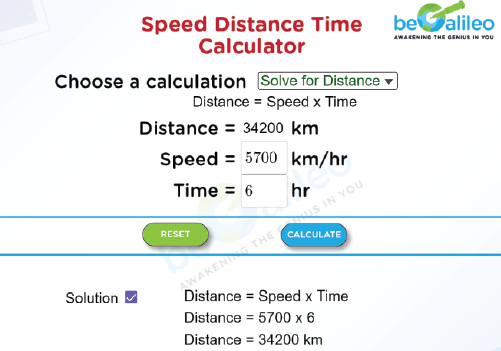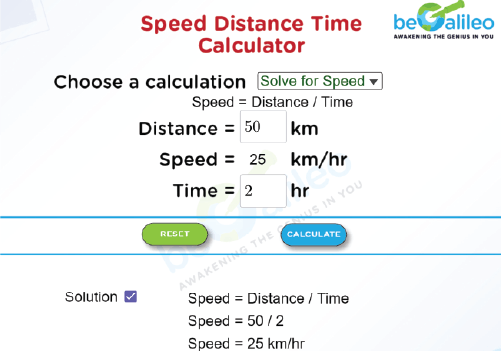>### Master Math with 1:1 Online Classes. Learn from Experts!# Speed Distance Time Calculator

The Speed, Distance, and Time Calculator, also referred to as an SDT Calculator, is an online calculator tool that is used for solving problems involving these three fundamental variables. This calculator makes it simple to determine a vehicle's average speed, distance traveled, and trip duration. In physics, this calculator can be used to analyze how objects move. This is also used in sports to evaluate an athlete's performance.

## How to use Speed, Distance, Time Calculator?

We can use the following steps in the calculator to find speed, distance, and time.

Steps Speed Distance Time
1 Select Solve for Speed in the drop down. Select Solve for Distance in the drop down. Select Solve for Time in the drop down.
2 Enter the distance in kilometres (km) and time in hours (hr). Enter the speed in kilometers per hour (km/hr) and time in hours (hr). Enter the distance in kilometres (km) and speed in kilometers per hour (km/hr).
3 Click on CALCULATE button
4 Speed will be displayed on the calculator. Distance will be displayed on the calculator. Time will be displayed on the calculator.
5 Select the Solution checkbox to view the detailed calculation.
6 Further, click the RESET button for the next calculation.

## How to find Speed, Distance and Time?

The Formulae used to find Speed, Distance and Time are,

• \text{Distance} =\text{Speed} \times \text{Time}
• \text{Speed} =\frac{\text{Distance}}{\text{Time}}
• \text{Time} =\frac{\text{Distance}}{\text{Speed}}

We need the other two in order to calculate one of the variables (time, speed, or distance).

Here, distance is measured in kilometers, time is measured in hours, and speed is measured in kilometers per hour.

## Solved Examples :

### Q1. What is the car's speed if it covers 66 km in two hours?

Solution :
Given, Distance = 66 km and Time = 2 hr
Speed= \frac{\text{Distance}}{\text{Time}}
Speed= \frac{66}{2}=33 km/hr
Hence, the speed of the car is 33 km/hr.### Q2. How long would it take a person to ride a bike at a speed of 13 km/hr for 855 kilometers?

Solution :
Given, Speed = 13 km/hr and Distance = 855 km
Time= \frac{\text{Distance}}{\text{Speed}}
Time= \frac{855}{13}=65.77 hr
Hence, time taken for the person is 65.77 hr.### Q3. What distance does a train cover if it travels at a speed of 5700 kilometers per hour for 6 hours?

Solution :
Given, Speed = 5700 km/hr and Time = 6 hr
\text{Distance = Speed} \times \text{Time}
\text{Distance = 5700} \times \text{6 = 34,200 km}
As a result, the train travels 34,200 km.### Q4. An athlete crosses a distance of 50 km in 2 hrs. What is his speed in km/hr?

Solution :
Given, Distance = 50 km and Time = 2 hr
Speed =\frac{\text{Distance}}{\text{Time}}
Speed =\frac{50}{2}=25 km/hr
Hence, the speed of the athlete is 25 km/hr.